Definitions of Square Dance Calls and Concepts

Index -->  Plus  |  A1  |  A2  |  C1  |  C2  |  C3A  |  C3B  |  C4  |  NOL  |
Definitions (Text Only) -->  Plus  |  A1  |  A2  |  C1  |  C2  |  C3A  |  C3B  |  C4  |  NOL  |
 Find call:

Split Phantom Columns | Lines | Waves Concept -- [C3A]
(Keith Gulley 1977)

\$BNY\$j9g\$C\$?(B Column | Line | Wave \$B\$,(B 4 \$B\$D8+\$D\$+\$k\$h\$&\$J(B 4 x 4 Matrix \$B\$+\$i!%(B

4 x 4 Matrix \$B\$rNY\$j9g\$C\$?(B 2 \$BAH\$N(B Column | Line | Wave \$B\$KJ,\$1(B, each side \$B\$G\$G\$-\$?(B 2 x 4 Matrix \$B\$GF0\$-\$^\$9!%(B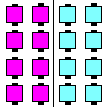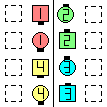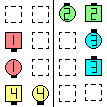Split Phantom Columns\$B\$G\$O\$3\$l\$i\$N(B position \$B\$GF0\$-\$^\$9(B Split Phantom ColumnsTriple Cross\$B\$NA0(B \$B8e(B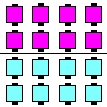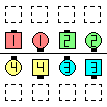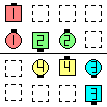Split Phantom Lines | Waves\$B\$G\$O\$3\$l\$i\$N(B position \$B\$GF0\$-\$^\$9(B Split Phantom WavesIn Roll Circulate\$B\$NA0(B \$B8e(B

\$BCm
• \$B\$b\$7M?\$(\$i\$l\$?%3!<%k\$G(B, phantom \$B\$N8~\$-\$rCN\$kI,MW\$,\$"\$k\$H\$-\$O(B, Split Phantom Columns | Lines | Waves formation \$B\$G(B phantom \$B\$N(B opposite \$B\$r8+\$F\$/\$@\$5\$\$!%\$=\$N>l=j\$K?M\$,\$\$\$l\$P(B, phantom \$B\$O\$=\$N?M\$N5U\$r8~\$\$\$F\$\$\$^\$9!%\$3\$l\$O(B Assume Symmetry (\$BLuN\$G\$"\$k\$3\$H\$r2>Dj\$9\$k(B) \$B\$H\$7\$FCN\$i\$l\$F\$\$\$^\$9!%\$"\$k%3!<%i!<\$O(B, \$B>o\$K\$3\$N\$h\$&\$K\$9\$Y\$-\$H?.\$8\$F\$\$\$^\$9\$,(B, \$BB>\$N%3!<%i!<\$O(B, \$B\$7\$F\$O\$\$\$1\$J\$\$\$H?.\$8\$F\$\$\$^\$9(B (\$B;d\$O8e
• \$B\$b\$7%3!<%i!<\$,(B Split Phantom Waves \$B\$H8@\$(\$P(B, Wave \$B\$G\$J\$1\$l\$P\$J\$i\$J\$\$\$N\$G(B, phantom \$B\$N8~\$-\$K4X\$7\$F\$N>pJs\$,\$"\$j\$^\$9!%(B
• Set \$B\$r(B 2 \$B\$D\$KJ,\$1\$???\$sCf\$K\$O(B, \$BN{4\$\$NJI(B (\$BLuJ,\$NJ}\$X9T\$C\$F\$7\$^\$&\$3\$H\$rK8\$2\$k\$G\$7\$g\$&!%(B
• Shape-change \$B\$9\$k%3!<%k\$,5v\$5\$l\$^\$9!%M?\$(\$i\$l\$?%3!<%k\$r\$7\$F(B, phantom \$B\$N9T\$-@h\$b4^\$a\$F(B, \$BI,MW\$J\$i7k2L\$N(B formation \$B\$,\$*8_\$\$\$KNY\$K\$J\$k\$h\$&\$K(B breathe \$B\$7\$^\$9!%(B
• \$BNr;KE*\$K\$O(B, Split Phantom Columns | Lines | Waves \$B\$O(B Phantom Split Columns | Lines | Waves \$B\$H\$b%3!<%k\$5\$l\$^\$7\$?!%(B
• T-Bone formation \$B\$+\$i(B, \$B%@%s%5!<\$O3F<+\$G(B 4 x 4 formation \$B\$r\$I\$N\$h\$&\$KJ,\$1\$k\$+\$r7h\$a\$^\$9!%(B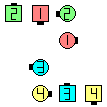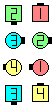Split Phantom WavesIn Roll Circulate\$B\$NA0(B \$B8e(B
\$B>e\$N%l%Y%k\$G\$O(B, Split Phantom Columns | Lines | Waves \$B\$O(B 1 x 16 \$B\$+\$i\$b;H\$o\$l\$^\$9!%(B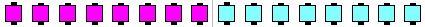Split Phantom Lines\$B\$G\$O\$3\$l\$i\$N(B position \$B\$GF0\$-\$^\$9(B

\$B

• Split Phantom Lines / Columns (by Charles Young)
•Choreography for Split Phantom Columns | Lines | Waves ConceptComments? Questions? Suggestions?

https://www.ceder.net/def/splitphantomclw.php?level=C3A&language=japan
26-July-2021 16:49:16